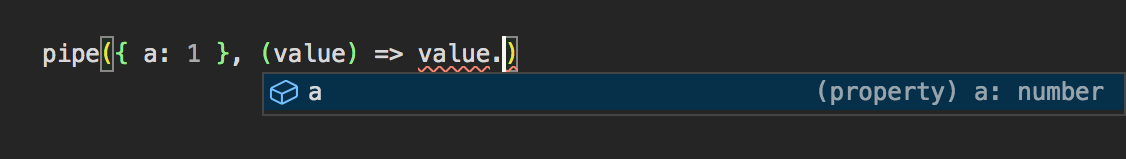# I've used the pipe() function 2,560 times and I can tell you it's good!

Posted on March 3, 2021 on Obvibase under the hood by Ivan Novikov.

The `pipe()` function that I'm talking about is the one that lets you replace `b(a(x))` with `pipe(x, a, b)`. Yes, that's how many times I've used it over the last few years, and looking back at those usages, I'd like to tell you the reasons you might find it useful too, even when you work with a codebase that doesn't stray from mainstream patterns into functional programming.

# Where it's coming from

`pipe` takes the first argument and pipes it though each of the functions that you provide as the remaining arguments, and can be implemented as follows:

``````const pipe = (x, ...fns) => fns.reduce((acc, el) => el(acc), x);
``````

You can type it in TypeScript using overloads, and since as far back as TypeScript 3.4, type inference works perfectly:One way to look at this function is to see it as a fill-in for the proposed pipeline operator (`x |> a |> b`). That proposal has been at stage 1 for years, but the good news is that `pipe` is not far worse — curiously, it's even better than some of the discussed flavors of the operator in one sense, namely that you don't have to enclose arrow functions in parens. If one of the flavors of the pipeline operator does reach stage 3, you won't be left out in the cold: with AST tools and Prettier, it would be easy to build a codemod that replaces `pipe` with the operator.

Putting aside the pipeline operator, `pipe` can be just seen as the first choice among different ways to do function composition. Another notable contender is a function that composes functions without applying them,

``````const ltrCompose = (...fns) => (x) => fns.reduce((acc, el) => fn(acc), x);
``````

so `b(a(x))` is equivalent to `ltrCompose(a, b)(x)`. It's a higher-order function though, and that's where `pipe` beats it: `pipe` is easier to read because it lets you achieve the same ends without thinking in terms of transforming functions to other functions. At first I tried using both utilities depending on the context, but I found this to be a bad violation of "only one way to do it".

# It's like dot-chaining

Now to reasons for using `pipe`. The first thing to notice is that rather than introducing a new pattern, `pipe` lets you use essentially the same pattern as dot-chaining,

``````yourArray.filter(predicate).map(project);
yourString.trim().toLowerCase();
``````

only without being constrained to the collection of methods defined for native objects.

One group of use-cases center around the fact that native JavaScript APIs were not designed with an eye to immutable updates that we often use today. `sort` method of `Array` and `add` method of `Set` are mutating, but with `pipe`, we can define their non-mutating counterparts

``````const sort = (compare) => (array) => [...array].sort(compare);

``````

and use them like we use dot-chained methods:

``````const newArray = pipe(array, sort(compare));
``````

Another common use-case is iterables. To take one example, if you need to filter values of a `Map`, you would have to write `[...yourMap.values()].filter(predicate)`, in other words, you have to convert the iterable returned by `yourMap.values` to an array just to get at the `filter` method. It wouldn't matter that much if it was just a question of performance, but it's both inefficient and clutters up the code. `pipe` gives you an alternative of working with iterables in the same way that you work with arrays:

``````const filter = (predicate) =>
function* (iterable) {
for (const el of iterable) {
if (predicate(el)) {
yield el;
}
}
};

const filteredValuesIterable = pipe(yourMap.values(), filter(predicate));
``````

# It lets you create locals with expressions

Here's another reason for using `pipe` — and this time we're not even going to need any utility functions other than `pipe` itself.

Imagine that in an `if` clause, you need to convert a string to a number and check if that number is greater than 0.

``````if (parseFloat(str) > 0) {
// ...
}
``````

Now suppose that we also need to check that the number is less than 1. Unless we want to duplicate `parseFloat` calls, we have to define a new constant in the outer scope:

``````const num = parseFloat(str);
if (num > 0 && num < 1) {
// ...
}
``````

Wouldn't it be better if `num` was scoped to the expression in the `if` clause, which is the only place where we need it? This can be accomplished with an IIFE, but it's not pretty:

``````if (
(() => {
const num = parseFloat(str);
return num > 0 && num < 1;
})()
) {
// ...
}
``````

`pipe` solves the problem:

``````if (pipe(str, parseFloat, (num) => num > 0 && num < 1)) {
// ...
}
``````

Generally speaking, in any context where an expression is expected, whether it's a function argument, an element in an array/object literal, or an operand of a ternary operator, `pipe` lets you create a local without resorting to IIFE. This tends to make you rely more on expressions,

``````const reducer = (state, action) =>
action.type === `incrementA`
? pipe(state, ({ a, ...rest }) => ({ ...rest, a: a + 1 }))
: action.type === `incrementB`
? pipe(state, ({ b, ...rest }) => ({ ...rest, b: b + 1 }))
: state;
``````

but you don't have to use expressions all the time — `pipe` just lets you make the choice between expressions and statements not based on syntax limitations, but based on what's more readable in a specific situation.

The `pipe` function as defined here is available in fp-ts. If like me you don't need a full-blown functional programming library, you can get `pipe` in my own library Antiutils.